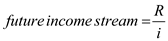# Quiz : Special Topic 4 Great Debates in Economics: Keynes Versus Hayek

The value of a stock depends on three factors: the expected future earnings, the maturity date and the present discount rate. The present discount rate depends on the market interest rate. The higher the interest rate the lower will be the value of future income and vice versa. If R is the annual revenue stream and i is the interest rate then the present value of the future income stream is given byOn the other hand, when future income from a stock will be higher the value of the stock will be higher. Hence, when the interest rate is low, the value of a stock will be high. The real interest rate is the inflation rate plus the nominal interest rate. If monetary policy leads to higher inflation and nominal interest rate, then the real interest rate will also be high. A higher real interest implies that the value of stocks will be low. Hence, if the monetary policy leads to higher nominal interest rate and inflation rate, the value of stock price is likely to fall.